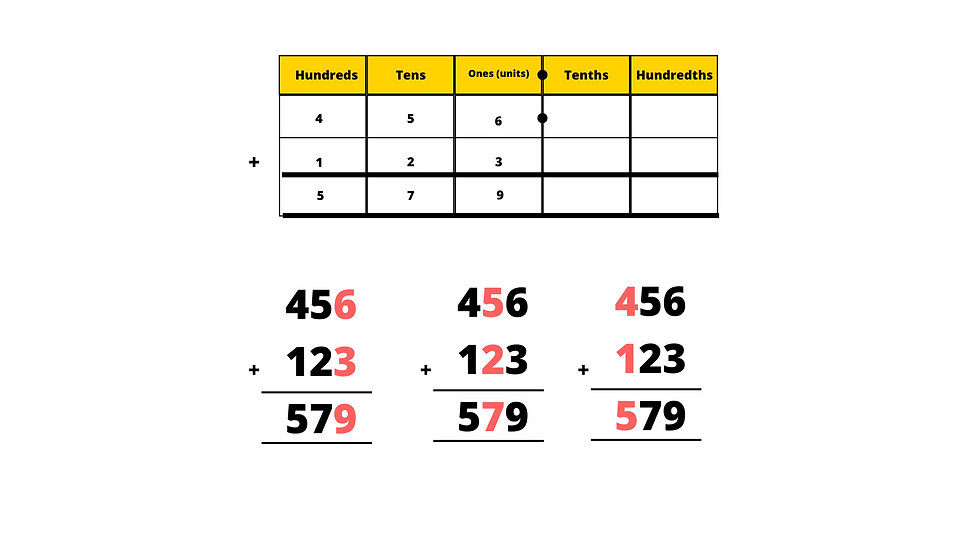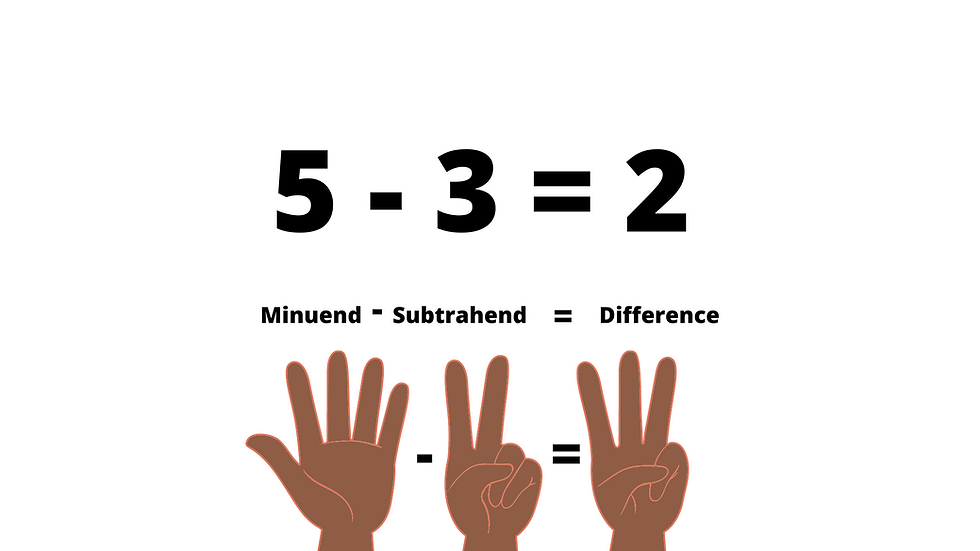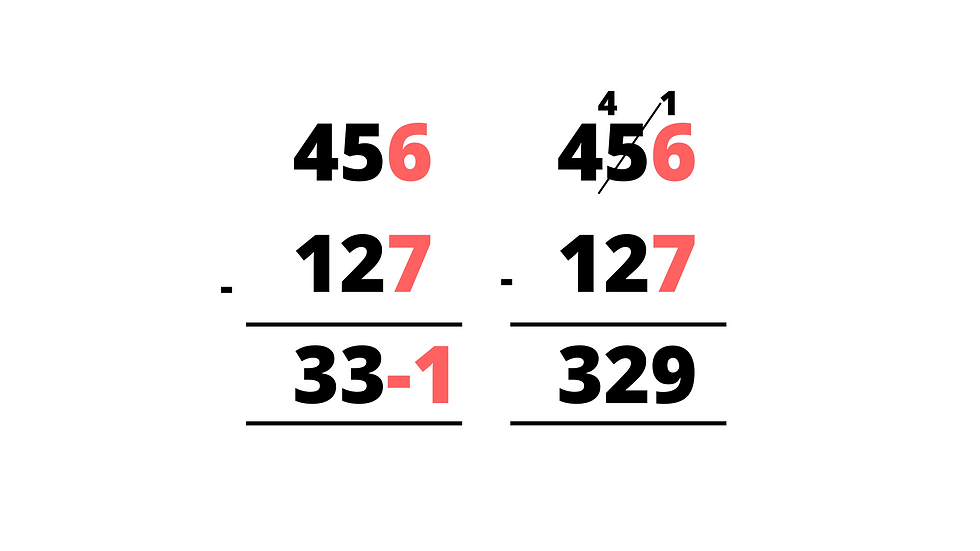top of page
Search

# How I wish I'd always taught... addition and subtraction.

Maths is full of symbols. Some of the more familiar are that addition and subtraction symbols. The equals symbol is a relative newcomer having been introduced in 1557 by Welsh mathematician Robert Recorde.

When we add, we sum together two (or more) quantities. This results in an increase in quantity (unless we are working with negative numbers, of course). Numbers being added together have useful names which can aid children to reason clearly. Let's take 9 + 1 = 10. 9 is the augend: the number in which is to be added to. 1 is the addend: the number too add to the augend. The total is 10 and so the sum is 10. As children gain fluency is adding 1, they begin to make links and connections known as number bonds which make patterns and can expose the structure of the number system.This may be the first time children look for structures and make generalisations in maths. They are useful to know as their recall aids fluency with arithmetic and understanding of the number system which is built around sets of 10 (including the decimal system).Laws

The three fundamental laws of addition unlock the structure of maths: associative law, commutative law and the distributive law. Understanding how the laws work leads to a deep understanding of how mathematics works.

Associative law.

This law states it does not matter how we group numbers with addition for the sum will always be the same. This law allows children to make calculations more efficient. For example:

9 + 12 + 8: it is more efficient to do 9 + (12 + 8) than (9 + 12) + 8. Number bonds to 20 help here too.

Commutative law

The commutative law states the order of addition makes no difference to the sum. For example: 1 + 2 = 2 + 1.

This is useful as children may encounter problems such as: 9 + 12 + 8 + 1. It would be much more efficient to look for number bonds such as 9 + 1 + 12 + 8 and achieving the same sum. We can express this algebraically: a + b = b + a.

Distributive law

This law requires numbers to be 'broken down' in small components. This is an essential skill for children to master to look for numbers within numbers. Essentially, looking for number bonds within a set of numbers. Let's look at this example:

27 + 14 + 11.

Here, we want to encourage children to apply the distributive law to break the problem down. It may look something like this:

• (20 + 7) + (10 + 4) + (10 + 1)

• (20 + 10+ 10) + (7 + 4 + 1)

• (40 + 12)

• = 52Column methods

The idea of breaking numbers down into smaller components (the distributive law) is an essential concept to mastery for mathematical success. Here, the column method of addition breaks (partitions) a number into its place value. Many teachers, parents and children will be familiar with this method.This method works by essentially transforming a calculation into (6 + 3) + (50 + 20) + (400 + 100). When we cross the tens boundary, we must carry to the greater place value section. Without carrying, calculations would look like this:Using place value counters can be an effective way to demonstrate how the column method works efficiently. The highlight ones in the sum, show that 10 ones are exchanged for 1 ten.Subtraction

Subtraction is the concept of reducing a number or quantity by another. It 's etymology lies in the latin for 'removal'.

Take the example: 5 - 2 = 3.

We can represent this in many different ways.Laws

Unlike addition, subtraction is not commutative and must be done in order (there are some exceptions to subtraction calculation with more than 2 values: 25 - 5 - 3 - 2) (This is because the subtrahends can be added together and addition is commutative).

Therefore 5 - 3 3 - 5.

As we saw with augend, addend and sum in addition, subtraction too has specific names for each part of a calculation. There is a good reason for this as it avoids ambiguity and confusion when reasoning.Associative

Subtraction is not associative because: (12 - 5) - 2 12 - (5 - 2). This means we would be changing the value of the subtrahend.

Same difference

Understanding that subtraction can be considered as finding the difference between two numbers and changing the subtrahend and minuend by the same amount, the difference will always remain the same.

For example: 10 - 8 = 2, 6 - 4 = 2, 5 - 3 = 2 and 3 - 1 = 2. The difference between the subtrahend and minuend are always 2. This can be useful for calculations such as: 33 - 7, changing the subtrahend and minuend so they have the same difference (36 - 10 = 26) allows for efficient use of this strategy.Column subtraction

Column subtraction is visually similar to column addition. We place each digit in its place value position and stack them up.However, the method needs a different approach when the digit in the subtrahend is greater than the corresponding digit in the minuend. I am sure many children revert to looking for the difference between two digits and placing this in the answer. In the example below, we can almost make sense of not exchanging (300 and 30 subtract 1 = 329). This however, does not use the decimal form.

Referring to each digit by its place value can support understanding here. Exchanging 1 ten from the 5 tens and placing the exchanged ten in the ones column creates 16 - 7.This can be avoided all together using the same difference concept. Using place value counters, in a similar way as we did this addition, can strengthen and deepen understanding of the column method of subtraction.